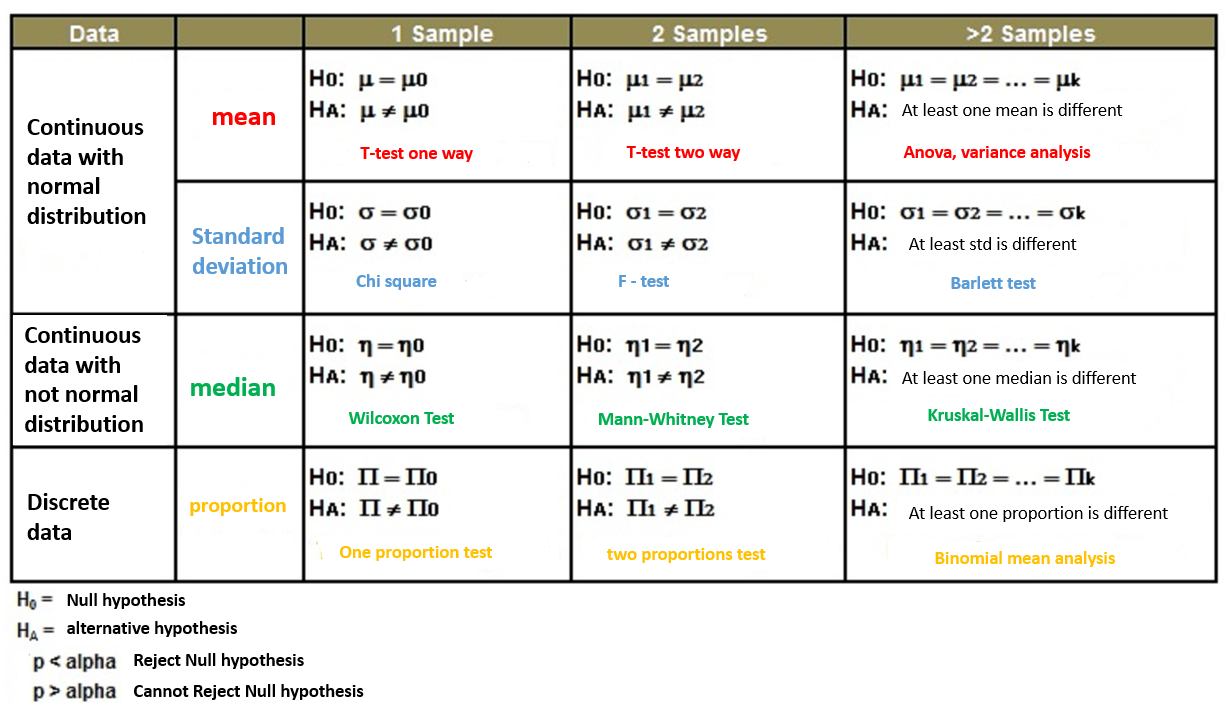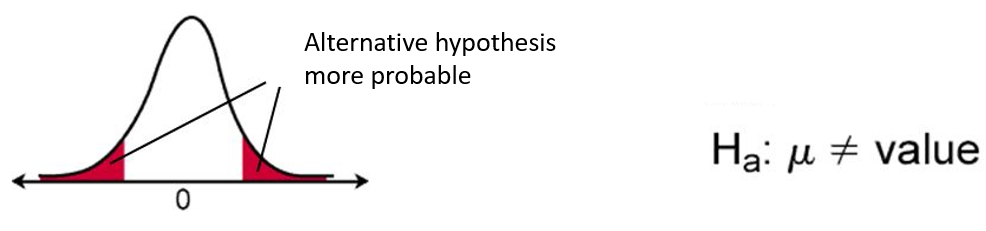hypo-eng

A statistical hypothesis is a statement about the distribution of a population. It may concern either its functional form (i.e. what is the distribution of the statistical population – the most frequently checked one is the normal distribution) or the parameter values (most often the arithmetic mean).
Examples of statistical hypotheses include: the average weight of women at the age of 30 is 65 kg; preschoolers watch TV on average 2 hours a day; Poles read an average of 2 books a year, etc.
There are two types of hypotheses as we have already mentioned:

• Nonparametric hypothesis – the assumption concerns the form of distribution of the population trait
• Parametric hypothesis – the assumption concerns the values of the parameters of the distribution of the population trait

Statistical test – a rule of conduct which assigns (with a set probability) to each possible trial implementation (x1, …, xn) the decision to accept or reject the tested hypothesis.

• Parametric test – refers to the parametric hypothesis
• Non-parametric test (compliance test) – refers to a non-parametric hypothesis
Examples of statistical tests:
from IPython.display import Image
Image(filename="img/hypo.png")The assumption of the statistical test is called the null hypothesis (H0). This is often called the default assumption or the assumption that nothing has changed. In contrast, a test assumption violation is often referred to as hypothesis 1 (H1) or an alternative hypothesis.

• Hypothesis 0 (H0): The test assumption is held up and cannot be rejected at some significance level.
• Hypothesis 1 (HA): The test assumption is rejected at some significance level.

The goals of formulating statistical hypotheses may be different. It all depends on the test, e.g.

• study of assumptions about the average level of a trait in the population – we check data on the average weight of women in the population,
• the difference between 2 groups – we check whether preschoolers in the city and preschoolers in the countryside watch the same amount of TV during the day
• testing the relationship between features – we verify whether reading more books depends on education
• comparison of variable distributions – most often we examine whether the observed feature has a normal or close to normal distribution

The key to success in statistical hypothesis testing is the correct formulation of the hypotheses. Most often, as the null hypothesis, we take the sentence that we want to reject, because the error of such a decision can be controlled. The logic of testing is as follows: we create a function of random variables, for which, if these variables satisfy the null hypothesis, we can give the probabilities with which it takes different values. Then we calculate the value of this function for the tested sample. If the probability of achieving the obtained or even more extreme value of the statistic is low, we doubt that our data is consistent with the null hypothesis and we are inclined to adopt an alternative hypothesis.

##### Test statistics¶

There are two common forms that can arise from a statistical hypothesis test and must be interpreted in different ways. These are p-values and critical values. A statistical hypothesis test can return a p-value. This is the amount that we can use to interpret or quantify the test result and either reject or not reject the null hypothesis. This is done by comparing the p-value with a pre-selected threshold value called the significance level. The significance level is often indicated by the Greek lowercase alpha. The most common value used for alpha is 5% or 0.05. A lower alpha value suggests a more reliable interpretation of the null hypothesis, e.g. 1% or 0.1%. The result is statistically significant when the p-value is less than alpha. This means that a change has been detected: the default hypothesis can be rejected.

-If p-value> alpha: You cannot reject the null hypothesis (ie, it is not a significant result).
-If p-value <= alpha: Reject the null hypothesis (ie, Significant result).

For example, if we tested whether a sample of the data was normal and we calculated the value p = 0.08 (p> alpha), we can conclude:

The test showed that the data sample was normal without rejecting the null hypothesis at the 5% significance level. This means that when we interpret the result of a statistical test, we don’t know what is true or false, only what is probable. In other words, we chose to reject or not to reject the null hypothesis at a certain level of statistical significance based on empirical evidence and the chosen statistical test.

Rejecting the null hypothesis means that there is sufficient statistical evidence that the null hypothesis does not look probable. Otherwise, it means that there is not enough statistical evidence to reject the null hypothesis.

Critical values is the area that is always at the margins of the distribution. If the value of the test statistic (p) calculated by us falls within this area, we reject the null hypothesis. The size of the critical area is determined by arbitrarily small α, while its position is determined by an alternative hypothesis.

Image(filename="img/hypo3.png")##### Statistical errors¶

Type I error – occurs when you reject the null hypothesis, and in fact it was true. So if our hypothesis was: preschoolers both in the city and in the countryside watch the same amount of television and we rejected this hypothesis in favor of the alternative hypothesis that there is a difference between preschoolers in the length of watching television, then we make a type I error. The probability of making a type I error when the null hypothesis was true is called the significance level (alpha), level indicates the maximum risk of the first type of error that we are willing to accept. The choice of α value depends on the test, most often it is 0.05 but it can also be 0.1 or 0.01. Suppose alpha is 5%, this suggests (at most) 1 time in 20 that the null hypothesis will be erroneously rejected or not rejected due to statistical noise in the data sample.

Type II error – occurs when we do not reject a false hypothesis. It takes place when, in fact, the time spent in front of the TV children from villages and cities differs, and we will not reject this hypothesis. We do not reject the null hypothesis, even though it is false. The probability of making mistakes of the second type is denoted by the symbol β (beta). The reduction of the second type of errors is very important in some tests. This is a much more serious error than Type I.

Image(filename="img/hypo2.png")